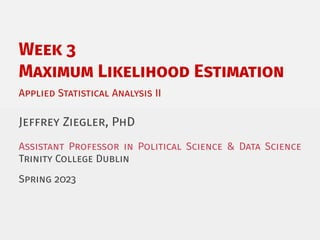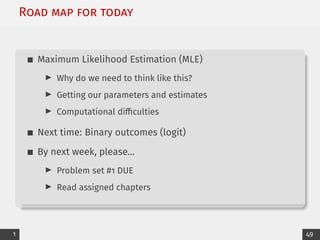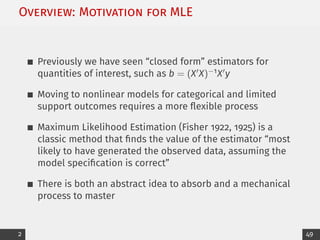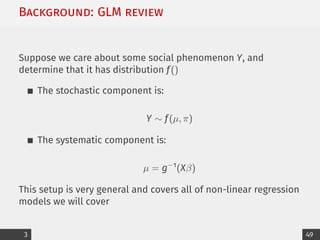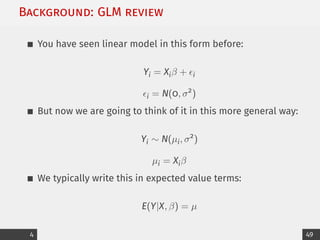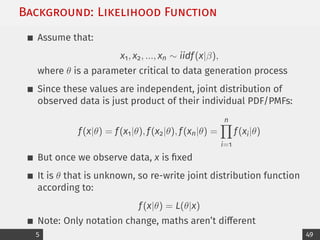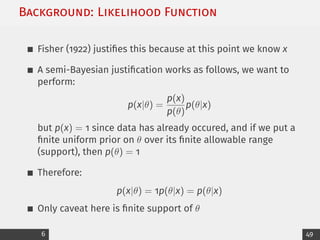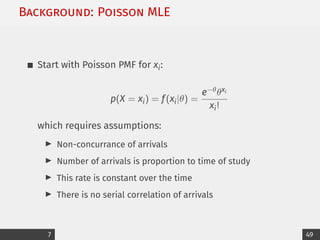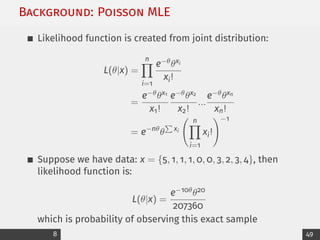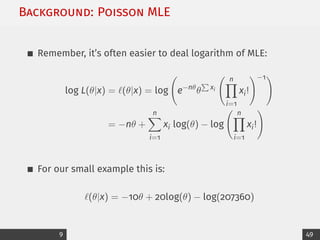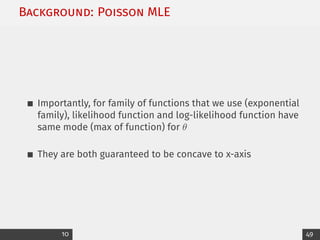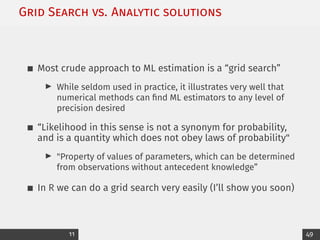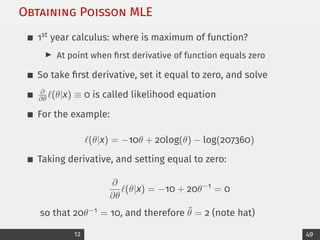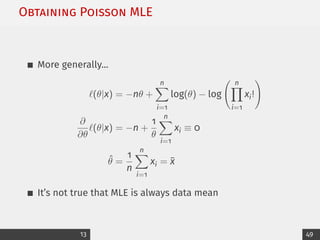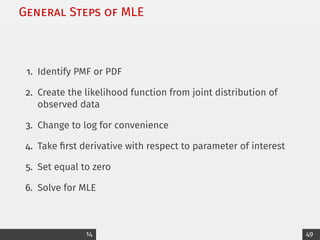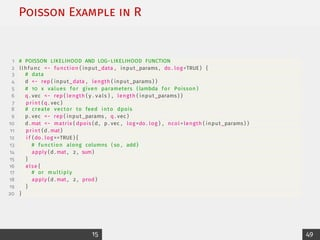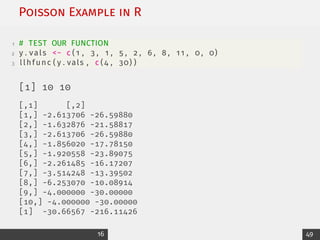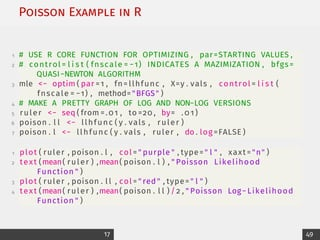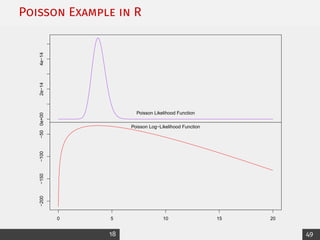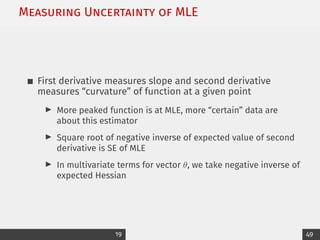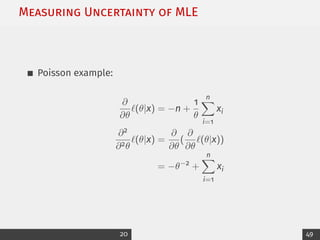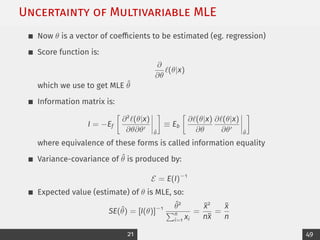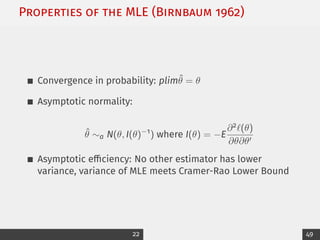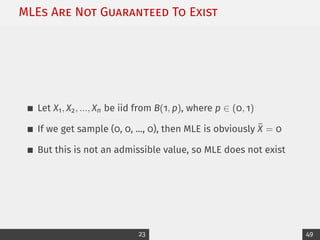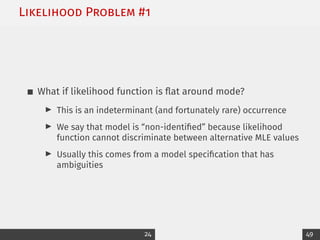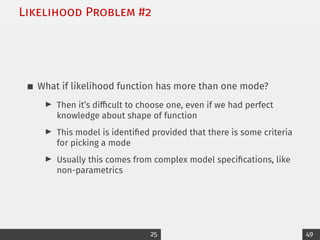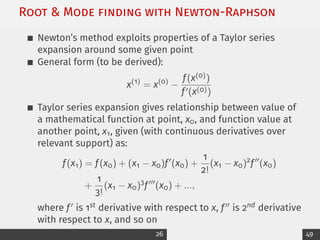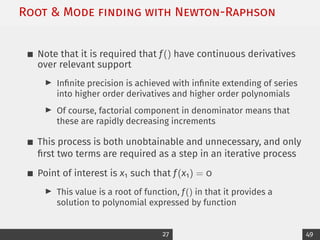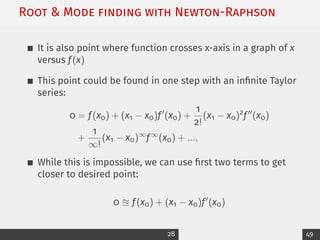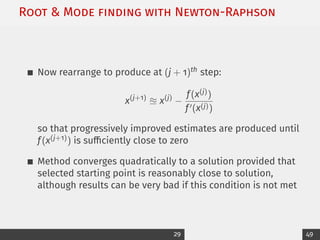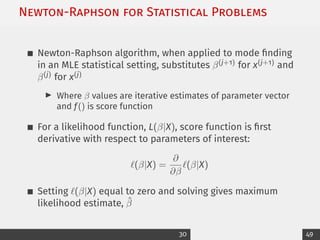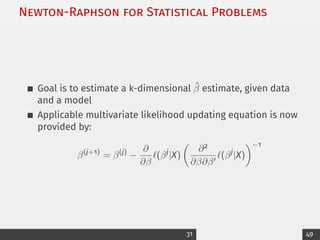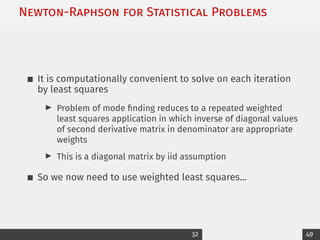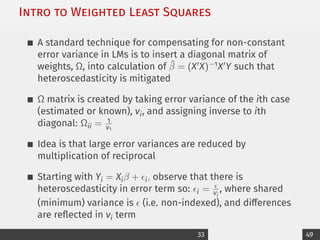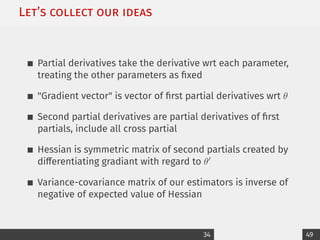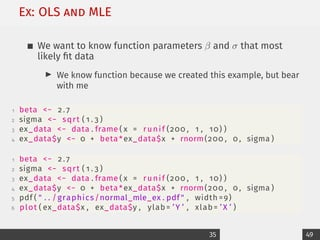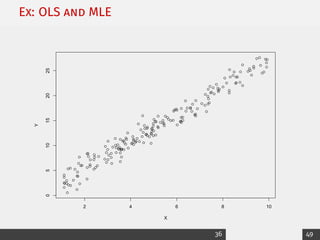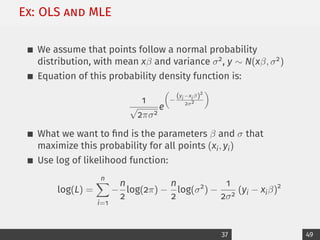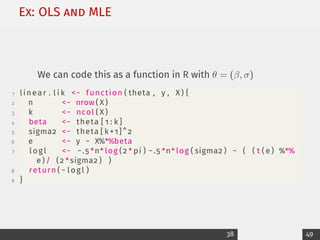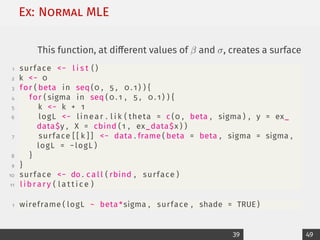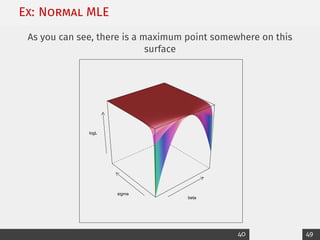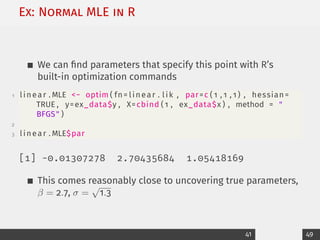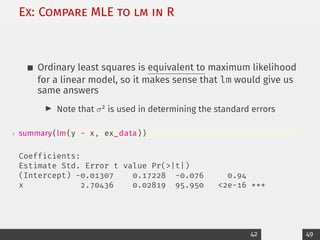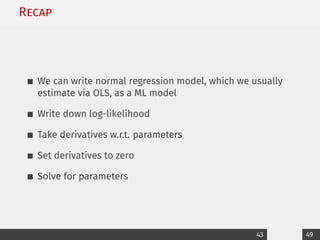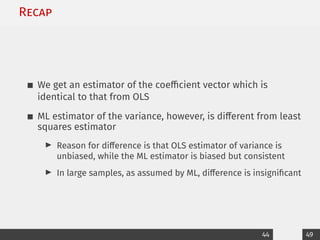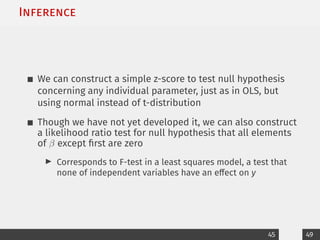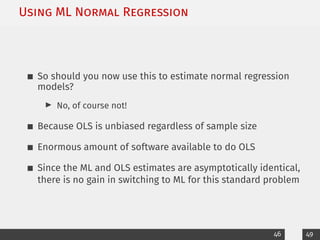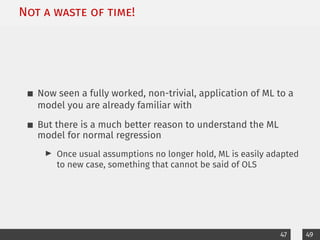1 von 50Matlab polynimials and curve fittingAmeen SanMachine learning (1)NYversityCS229 Machine Learning Lecture NotesEric ConnerLecture 1 maximum likelihoodAnant DashputeConditional Random FieldslswingIntroduction to Bootstrap and elements of Markov ChainsUniversity of Salerno

### 3_MLE_printable.pdf

1. Week 3 Maximum Likelihood Estimation Applied Statistical Analysis II Jeffrey Ziegler, PhD Assistant Professor in Political Science & Data Science Trinity College Dublin Spring 2023
2. Road map for today Maximum Likelihood Estimation (MLE) I Why do we need to think like this? I Getting our parameters and estimates I Computational difficulties Next time: Binary outcomes (logit) By next week, please... I Problem set #1 DUE I Read assigned chapters 1 49
3. Overview: Motivation for MLE Previously we have seen “closed form” estimators for quantities of interest, such as b = (X0X)−1X0y Moving to nonlinear models for categorical and limited support outcomes requires a more flexible process Maximum Likelihood Estimation (Fisher 1922, 1925) is a classic method that finds the value of the estimator “most likely to have generated the observed data, assuming the model specification is correct” There is both an abstract idea to absorb and a mechanical process to master 2 49
4. Background: GLM review Suppose we care about some social phenomenon Y, and determine that it has distribution f() The stochastic component is: Y ∼ f(µ, π) The systematic component is: µ = g−1 (Xβ) This setup is very general and covers all of non-linear regression models we will cover 3 49
5. Background: GLM review You have seen linear model in this form before: Yi = Xiβ + i i = N(0, σ2 ) But now we are going to think of it in this more general way: Yi ∼ N(µi, σ2 ) µi = Xiβ We typically write this in expected value terms: E(Y|X, β) = µ 4 49
6. Background: Likelihood Function Assume that: x1, x2, ..., xn ∼ iidf(x|β), where θ is a parameter critical to data generation process Since these values are independent, joint distribution of observed data is just product of their individual PDF/PMFs: f(x|θ) = f(x1|θ), f(x2|θ), f(xn|θ) = n Y i=1 f(xi|θ) But once we observe data, x is fixed It is θ that is unknown, so re-write joint distribution function according to: f(x|θ) = L(θ|x) Note: Only notation change, maths aren’t different 5 49
7. Background: Likelihood Function Fisher (1922) justifies this because at this point we know x A semi-Bayesian justification works as follows, we want to perform: p(x|θ) = p(x) p(θ) p(θ|x) but p(x) = 1 since data has already occured, and if we put a finite uniform prior on θ over its finite allowable range (support), then p(θ) = 1 Therefore: p(x|θ) = 1p(θ|x) = p(θ|x) Only caveat here is finite support of θ 6 49
8. Background: Poisson MLE Start with Poisson PMF for xi: p(X = xi) = f(xi|θ) = e−θθxi xi! which requires assumptions: I Non-concurrance of arrivals I Number of arrivals is proportion to time of study I This rate is constant over the time I There is no serial correlation of arrivals 7 49
9. Background: Poisson MLE Likelihood function is created from joint distribution: L(θ|x) = n Y i=1 e−θθxi xi! = e−θθx1 x1! e−θθx2 x2! ... e−θθxn xn! = e−nθ θ P xi n Y i=1 xi! !−1 Suppose we have data: x = {5, 1, 1, 1, 0, 0, 3, 2, 3, 4}, then likelihood function is: L(θ|x) = e−10θθ20 207360 which is probability of observing this exact sample 8 49
10. Background: Poisson MLE Remember, it’s often easier to deal logarithm of MLE: log L(θ|x) = (θ|x) = log e−nθ θ P xi n Y i=1 xi! !−1! = −nθ + n X i=1 xi log(θ) − log n Y i=1 xi! ! For our small example this is: (θ|x) = −10θ + 20log(θ) − log(207360) 9 49
11. Background: Poisson MLE Importantly, for family of functions that we use (exponential family), likelihood function and log-likelihood function have same mode (max of function) for θ They are both guaranteed to be concave to x-axis 10 49
12. Grid Search vs. Analytic solutions Most crude approach to ML estimation is a “grid search” I While seldom used in practice, it illustrates very well that numerical methods can find ML estimators to any level of precision desired “Likelihood in this sense is not a synonym for probability, and is a quantity which does not obey laws of probability I Property of values of parameters, which can be determined from observations without antecedent knowledge” In R we can do a grid search very easily (I’ll show you soon) 11 49
13. Obtaining Poisson MLE 1st year calculus: where is maximum of function? I At point when first derivative of function equals zero So take first derivative, set it equal to zero, and solve ∂ ∂θ (θ|x) ≡ 0 is called likelihood equation For the example: (θ|x) = −10θ + 20log(θ) − log(207360) Taking derivative, and setting equal to zero: ∂ ∂θ (θ|x) = −10 + 20θ−1 = 0 so that 20θ−1 = 10, and therefore θ̂ = 2 (note hat) 12 49
14. Obtaining Poisson MLE More generally... (θ|x) = −nθ + n X i=1 log(θ) − log n Y i=1 xi! ! ∂ ∂θ (θ|x) = −n + 1 θ n X i=1 xi ≡ 0 θ̂ = 1 n n X i=1 xi = x̄ It’s not true that MLE is always data mean 13 49
15. General Steps of MLE 1. Identify PMF or PDF 2. Create the likelihood function from joint distribution of observed data 3. Change to log for convenience 4. Take first derivative with respect to parameter of interest 5. Set equal to zero 6. Solve for MLE 14 49
16. Poisson Example in R 1 # POISSON LIKELIHOOD AND LOG−LIKELIHOOD FUNCTION 2 llhfunc − function ( input_data , input_params , do . log =TRUE ) { 3 # data 4 d − rep ( input_data , length ( input_params ) ) 5 # 10 x values for given parameters ( lambda for Poisson ) 6 q . vec − rep ( length ( y . vals ) , length ( input_params ) ) 7 print (q . vec ) 8 # create vector to feed into dpois 9 p . vec − rep ( input_params , q . vec ) 10 d . mat − matrix ( dpois (d , p . vec , log=do . log ) , ncol= length ( input_params ) ) 11 print (d . mat) 12 i f (do . log ==TRUE ) { 13 # function along columns ( so , add ) 14 apply (d . mat , 2 , sum) 15 } 16 else { 17 # or multiply 18 apply (d . mat , 2 , prod ) 19 } 20 } 15 49
17. Poisson Example in R 1 # TEST OUR FUNCTION 2 y . vals − c ( 1 , 3 , 1 , 5 , 2 , 6 , 8 , 11 , 0 , 0) 3 llhfunc ( y . vals , c (4 , 30) )  10 10 [,1] [,2] [1,] -2.613706 -26.59880 [2,] -1.632876 -21.58817 [3,] -2.613706 -26.59880 [4,] -1.856020 -17.78150 [5,] -1.920558 -23.89075 [6,] -2.261485 -16.17207 [7,] -3.514248 -13.39502 [8,] -6.253070 -10.08914 [9,] -4.000000 -30.00000 [10,] -4.000000 -30.00000  -30.66567 -216.11426 16 49
18. Poisson Example in R 1 # USE R CORE FUNCTION FOR OPTIMIZING , par=STARTING VALUES , 2 # control = l i s t ( fnscale = −1) INDICATES A MAZIMIZATION , bfgs= QUASI−NEWTON ALGORITHM 3 mle − optim ( par =1 , fn= llhfunc , X=y . vals , control = l i s t ( fnscale = −1) , method=BFGS ) 4 # MAKE A PRETTY GRAPH OF LOG AND NON−LOG VERSIONS 5 ruler − seq ( from =.01 , to =20 , by= . 0 1 ) 6 poison . l l − llhfunc ( y . vals , ruler ) 7 poison . l − llhfunc ( y . vals , ruler , do . log =FALSE ) 1 plot ( ruler , poison . l , col = purple , type= l , xaxt = n ) 2 text (mean( ruler ) ,mean( poison . l ) , Poisson Likelihood Function ) 3 plot ( ruler , poison . l l , col = red , type= l ) 4 text (mean( ruler ) ,mean( poison . l l ) / 2 , Poisson Log− Likelihood Function ) 17 49
19. Poisson Example in R 0e+00 2e−14 4e−14 ruler poison.l Poisson Likelihood Function 0 5 10 15 20 −200 −150 −100 −50 ruler poison.ll Poisson Log−Likelihood Function 18 49
20. Measuring Uncertainty of MLE First derivative measures slope and second derivative measures “curvature” of function at a given point I More peaked function is at MLE, more “certain” data are about this estimator I Square root of negative inverse of expected value of second derivative is SE of MLE I In multivariate terms for vector θ, we take negative inverse of expected Hessian 19 49
21. Measuring Uncertainty of MLE Poisson example: ∂ ∂θ (θ|x) = −n + 1 θ n X i=1 xi ∂2 ∂2θ (θ|x) = ∂ ∂θ ( ∂ ∂θ (θ|x)) = −θ−2 + n X i=1 xi 20 49
22. Uncertainty of Multivariable MLE Now θ is a vector of coefficients to be estimated (eg. regression) Score function is: ∂ ∂θ (θ|x) which we use to get MLE θ̂ Information matrix is: I = −Ef ∂2 (θ|x) ∂θ∂θ0 θ̂ ≡ Eb ∂(θ|x) ∂θ ∂(θ|x) ∂θ0 θ̂ where equivalence of these forms is called information equality Variance-covariance of θ̂ is produced by: E = E(I)−1 Expected value (estimate) of θ is MLE, so: SE(θ̂) = [I(θ)]−1 θ̂2 Pn i=1 xi = x̄2 nx̄ = x̄ n 21 49
23. Properties of the MLE (Birnbaum 1962) Convergence in probability: plimθ̂ = θ Asymptotic normality: θ̂ ∼a N(θ, I(θ)−1 ) where I(θ) = −E ∂2(θ) ∂θ∂θ0 Asymptotic efficiency: No other estimator has lower variance, variance of MLE meets Cramer-Rao Lower Bound 22 49
24. MLEs Are Not Guaranteed To Exist Let X1, X2, ..., Xn be iid from B(1, p), where p ∈ (0, 1) If we get sample (0, 0, ..., 0), then MLE is obviously X̄ = 0 But this is not an admissible value, so MLE does not exist 23 49
25. Likelihood Problem #1 What if likelihood function is flat around mode? I This is an indeterminant (and fortunately rare) occurrence I We say that model is “non-identified” because likelihood function cannot discriminate between alternative MLE values I Usually this comes from a model specification that has ambiguities 24 49
26. Likelihood Problem #2 What if likelihood function has more than one mode? I Then it’s difficult to choose one, even if we had perfect knowledge about shape of function I This model is identified provided that there is some criteria for picking a mode I Usually this comes from complex model specifications, like non-parametrics 25 49
27. Root Mode ﬁnding with Newton-Raphson Newton’s method exploits properties of a Taylor series expansion around some given point General form (to be derived): x(1) = x(0) − f(x(0)) f0(x(0)) Taylor series expansion gives relationship between value of a mathematical function at point, x0, and function value at another point, x1, given (with continuous derivatives over relevant support) as: f(x1) = f(x0) + (x1 − x0)f0 (x0) + 1 2! (x1 − x0)2 f00 (x0) + 1 3! (x1 − x0)3 f000 (x0) + ..., where f0 is 1st derivative with respect to x, f00 is 2nd derivative with respect to x, and so on 26 49
28. Root Mode ﬁnding with Newton-Raphson Note that it is required that f() have continuous derivatives over relevant support I Infinite precision is achieved with infinite extending of series into higher order derivatives and higher order polynomials I Of course, factorial component in denominator means that these are rapidly decreasing increments This process is both unobtainable and unnecessary, and only first two terms are required as a step in an iterative process Point of interest is x1 such that f(x1) = 0 I This value is a root of function, f() in that it provides a solution to polynomial expressed by function 27 49
29. Root Mode ﬁnding with Newton-Raphson It is also point where function crosses x-axis in a graph of x versus f(x) This point could be found in one step with an infinite Taylor series: 0 = f(x0) + (x1 − x0)f0 (x0) + 1 2! (x1 − x0)2 f00 (x0) + 1 ∞! (x1 − x0)∞ f∞ (x0) + ..., While this is impossible, we can use first two terms to get closer to desired point: 0 u f(x0) + (x1 − x0)f0 (x0) 28 49
30. Root Mode ﬁnding with Newton-Raphson Now rearrange to produce at (j + 1)th step: x(j+1) u x(j) − f(x(j)) f0(x(j)) so that progressively improved estimates are produced until f(x(j+1)) is sufficiently close to zero Method converges quadratically to a solution provided that selected starting point is reasonably close to solution, although results can be very bad if this condition is not met 29 49
31. Newton-Raphson for Statistical Problems Newton-Raphson algorithm, when applied to mode finding in an MLE statistical setting, substitutes β(j+1) for x(j+1) and β(j) for x(j) I Where β values are iterative estimates of parameter vector and f() is score function For a likelihood function, L(β|X), score function is first derivative with respect to parameters of interest: (β|X) = ∂ ∂β (β|X) Setting (β|X) equal to zero and solving gives maximum likelihood estimate, β̂ 30 49
32. Newton-Raphson for Statistical Problems Goal is to estimate a k-dimensional β̂ estimate, given data and a model Applicable multivariate likelihood updating equation is now provided by: β(j+1) = β(j) − ∂ ∂β (βj |X) ∂2 ∂β∂β0 (βj |X) −1 31 49
33. Newton-Raphson for Statistical Problems It is computationally convenient to solve on each iteration by least squares I Problem of mode finding reduces to a repeated weighted least squares application in which inverse of diagonal values of second derivative matrix in denominator are appropriate weights I This is a diagonal matrix by iid assumption So we now need to use weighted least squares... 32 49
34. Intro to Weighted Least Squares A standard technique for compensating for non-constant error variance in LMs is to insert a diagonal matrix of weights, Ω, into calculation of β̂ = (X0X)−1X0Y such that heteroscedasticity is mitigated Ω matrix is created by taking error variance of the ith case (estimated or known), vi, and assigning inverse to ith diagonal: Ωii = 1 v1 Idea is that large error variances are reduced by multiplication of reciprocal Starting with Yi = Xiβ + i, observe that there is heteroscedasticity in error term so: i = vi , where shared (minimum) variance is (i.e. non-indexed), and differences are reflected in vi term 33 49
35. Let’s collect our ideas Partial derivatives take the derivative wrt each parameter, treating the other parameters as fixed Gradient vector is vector of first partial derivatives wrt θ Second partial derivatives are partial derivatives of first partials, include all cross partial Hessian is symmetric matrix of second partials created by differentiating gradiant with regard to θ0 Variance-covariance matrix of our estimators is inverse of negative of expected value of Hessian 34 49
36. Ex: OLS and MLE We want to know function parameters β and σ that most likely fit data I We know function because we created this example, but bear with me 1 beta − 2 . 7 2 sigma − sqrt ( 1 . 3 ) 3 ex_data − data . frame ( x = runif (200 , 1 , 10) ) 4 ex_data$y − 0 + beta *ex_data$x + rnorm(200 , 0 , sigma ) 1 beta − 2 . 7 2 sigma − sqrt ( 1 . 3 ) 3 ex_data − data . frame ( x = runif (200 , 1 , 10) ) 4 ex_data$y − 0 + beta *ex_data$x + rnorm(200 , 0 , sigma ) 5 pdf ( . . /graphics/normal_mle_ex . pdf , width =9) 6 plot ( ex_data$x , ex_data$y , ylab= ’Y ’ , xlab= ’ X ’ ) 35 49
37. Ex: OLS and MLE 2 4 6 8 10 0 5 10 15 20 25 X Y 36 49
38. Ex: OLS and MLE We assume that points follow a normal probability distribution, with mean xβ and variance σ2, y ∼ N(xβ, σ2) Equation of this probability density function is: 1 √ 2πσ2 e − (yi−xiβ)2 2σ2 What we want to find is the parameters β and σ that maximize this probability for all points (xi, yi) Use log of likelihood function: log(L) = n X i=1 − n 2 log(2π) − n 2 log(σ2 ) − 1 2σ2 (yi − xiβ)2 37 49
39. Ex: OLS and MLE We can code this as a function in R with θ = (β, σ) 1 linear . l i k − function ( theta , y , X ) { 2 n − nrow ( X ) 3 k − ncol ( X ) 4 beta − theta [ 1 : k ] 5 sigma2 − theta [ k +1]^2 6 e − y − X%*%beta 7 logl − −.5 *n* log (2 * pi ) −.5 *n* log ( sigma2 ) − ( ( t ( e ) %*% e ) / (2 *sigma2 ) ) 8 return ( − logl ) 9 } 38 49
40. Ex: Normal MLE This function, at different values of β and σ, creates a surface 1 surface − l i s t ( ) 2 k − 0 3 for ( beta in seq (0 , 5 , 0 . 1 ) ) { 4 for ( sigma in seq ( 0 . 1 , 5 , 0 . 1 ) ) { 5 k − k + 1 6 logL − linear . l i k ( theta = c (0 , beta , sigma ) , y = ex_ data$y , X = cbind ( 1 , ex_data$x ) ) 7 surface [ [ k ] ] − data . frame ( beta = beta , sigma = sigma , logL = −logL ) 8 } 9 } 10 surface − do . c a l l ( rbind , surface ) 11 l i b r a r y ( l a t t i c e ) 1 wireframe ( logL ~ beta *sigma , surface , shade = TRUE ) 39 49
41. Ex: Normal MLE As you can see, there is a maximum point somewhere on this surface beta sigma logL 40 49
42. Ex: Normal MLE in R We can find parameters that specify this point with R’s built-in optimization commands 1 linear . MLE − optim ( fn= linear . lik , par=c ( 1 , 1 , 1 ) , hessian= TRUE , y=ex_data$y , X=cbind ( 1 , ex_data$x ) , method = BFGS ) 2 3 linear . MLE\$par  -0.01307278 2.70435684 1.05418169 This comes reasonably close to uncovering true parameters, β = 2.7, σ = √ 1.3 41 49
43. Ex: Compare MLE to lm in R Ordinary least squares is equivalent to maximum likelihood for a linear model, so it makes sense that lm would give us same answers I Note that σ2 is used in determining the standard errors 1 summary( lm ( y ~ x , ex_data ) ) Coefficients: Estimate Std. Error t value Pr(|t|) (Intercept) -0.01307 0.17228 -0.076 0.94 x 2.70436 0.02819 95.950 2e-16 *** 42 49
44. Recap We can write normal regression model, which we usually estimate via OLS, as a ML model Write down log-likelihood Take derivatives w.r.t. parameters Set derivatives to zero Solve for parameters 43 49
45. Recap We get an estimator of the coefficient vector which is identical to that from OLS ML estimator of the variance, however, is different from least squares estimator I Reason for difference is that OLS estimator of variance is unbiased, while the ML estimator is biased but consistent I In large samples, as assumed by ML, difference is insignificant 44 49
46. Inference We can construct a simple z-score to test null hypothesis concerning any individual parameter, just as in OLS, but using normal instead of t-distribution Though we have not yet developed it, we can also construct a likelihood ratio test for null hypothesis that all elements of β except first are zero I Corresponds to F-test in a least squares model, a test that none of independent variables have an effect on y 45 49
47. Using ML Normal Regression So should you now use this to estimate normal regression models? I No, of course not! Because OLS is unbiased regardless of sample size Enormous amount of software available to do OLS Since the ML and OLS estimates are asymptotically identical, there is no gain in switching to ML for this standard problem 46 49
48. Not a waste of time! Now seen a fully worked, non-trivial, application of ML to a model you are already familiar with But there is a much better reason to understand the ML model for normal regression I Once usual assumptions no longer hold, ML is easily adapted to new case, something that cannot be said of OLS 47 49
49. Next week Specific GLMs: Binary outcomes 48 49
50. Class business Read required (and suggested) online materials Turn in Problem set # 1 before next class! 49 / 49